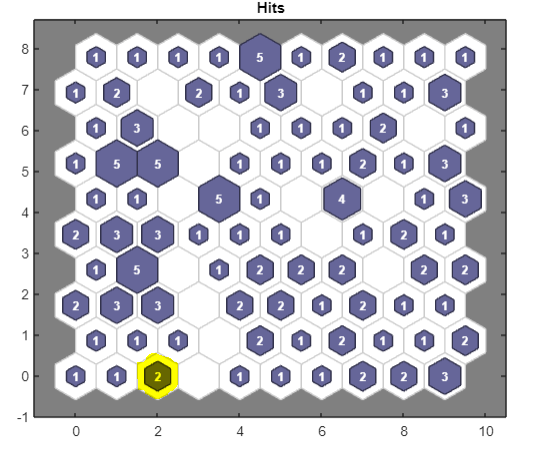# How to get input values from SOM sample hits plot in Matlab?Published

onThe self-organizing map (SOM) sample hits plot in the Matlab  shows the number of inputs in the neurons but one would be interested in knowing what these input values are. In this article, we are going to get the input values from the neurons.

Let’s say we have a sample hits plot (Figure 1) and we want to know the input values of the 3rd neuron (highlighted with yellow).  The numbering of neurons starts from bottom left to right. Here, the 3rd neuron shows 2 input values.Figure 1 Sample hits plot from Matlab .

Now, after you train your data, you have to add one command to convert vectors to indices.

For example, the following code performs SOM analysis on your data.

```>> x = data;
>> dimension1 = 10;
>> dimension2 = 10;
>> net = selforgmap([dimension1 dimension2]);
>> [net,tr] = train(net,x);
>> y = net(x);
% ---------Convert vector to indices----------------------
>> input_neuron_mapping = vec2ind(net(x))';```

vec2ind function will convert vectors to indices. ‘input_neuron_mapping’ is a vector now.
Now, if you want to see the input values of neuron 3, then first see the inputs by which neuron 3 was classified.

`>> neuron_3_input_indices = find(input_neuron_mapping == 3)`

Now, see the original input values.

```>> neuron_3_input_values = x(neuron_3_input_indices)
```

It will display 3 input values.

#### References

1. MATLAB. (2022). version 9.12.0 (R2022a). Natick, Massachusetts: The MathWorks Inc.Dr. Muniba is a Bioinformatician based in New Delhi, India. She has completed her PhD in Bioinformatics from South China University of Technology, Guangzhou, China. She has cutting edge knowledge of bioinformatics tools, algorithms, and drug designing. When she is not reading she is found enjoying with the family. Know more about Muniba

Click to comment

# How to define features/predictors of user dataset for SOM analysis in MATLAB?Published

onMost of the examples that demonstrate the SOM analysis use the default Iris dataset. It is also mentioned in the SOM example of MATLAB that for the user dataset, the predictors need to be defined . But it is not quite clear how to define the features or predictors of our dataset. In this article, we will learn how to define predictors for user datasets. (more…)